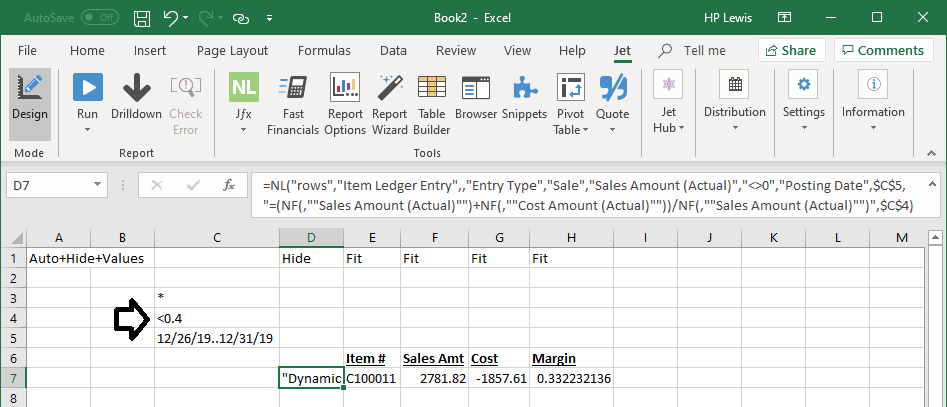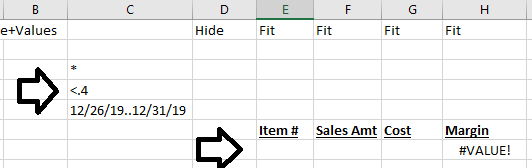Hi:

I tried running a calculation formula as a filter.  The report runs but does not produce any results - i know there should be results.  Here is my statement:

=NL("Rows","Item Ledger Entry","Item No.","Item No.",\$C\$3,"=(NF(,""Sales Amount (Actual)"")+NF(,""Cost Amount (Actual)""))/NF(,""Sales Amount (Actual)"")",\$C\$4,"Posting Date",\$C\$5,"Entry Type","Sale","Sales Amount (Actual)","<>0")

C4 is an amount ie:  <.2 - this is a below margin formula looking for transactions below margin.  Is this possible?  Or is there a better way to accomplish this?  Any help would be appreciated!

thanks,  Laura

•Harry Lewis

Hi Laura -

You may want to ensure that cell C4 is set to <0.2•Laura Porter

Thanks Harry!  This definitely worked!  But I have a follow up question... Is it possible to make that filter a Sum?  this part

"=(NF(,""Sales Amount (Actual)"")+NF(,""Cost Amount (Actual)""))/NF(,""Sales Amount (Actual)"")",\$C\$4,"Posting Date",\$C\$5,"Entry Type","Sale","Sales Amount (Actual)","<>0"

The problem with my statement is that it is looking at individual transactions and not the sum of all transactions for that period.  Is this even possible?

•Harry Lewis

You can.

You've got most of what you need, already.  I assume what you want is:

Instead of showing all transactions from the Item Ledger Entry table, you want to show each Item number from that that where SUM(Sales Amount - Cost Amount) / SUM(Sales Amount) is unequal to zero (or greater than or less than or some other number).

This involves the same concept as the Sort by Sum with Dynamics NAV.

I hope that helps.

-Harry

`Reports Your Way`
•Laura Porter

Thanks Harry :)   This is what I came up with but when I tried to get it in quotes it says "invalid field" when I try to run it.

="NL(""Sum"",""Item Ledger Entry"",""=(NF(,""Sales Amount (Actual)"")+NF(,""Cost Amount (Actual)""))/NF(,""Sales Amount (Actual)"")<"""&\$C\$4&""""",""Entry Type"",""Sale"",""Sales Amount (Actual)"",""<>0"",""Posting Date"","""&\$C\$5&""",""Item No."","""&\$C\$3&""")"

I did get a 0 value before I put it in quotes.  And I used the Sort by Sum method of \$C\$10 "*" for the filter in the item statement.  Not sure what I did wrong :(

•Laura Porter

Also i tried changing Item No. filter to NF(, "Item No.").

="NL(""Sum"",""Item Ledger Entry"",""=(NF(,""Sales Amount (Actual)"")+NF(,""Cost Amount (Actual)""))/NF(,""Sales Amount (Actual)"")<"""&\$C\$4&""""",""Entry Type"",""Sale"",""Sales Amount (Actual)"",""<>0"",""Posting Date"","""&\$C\$5&""",""Item No."",NF(,""Item No.""))"

Still get an Invalid Field :(

•Laura Porter

Update how silly of me -- missing the = sign at the beginning of statement!  Working now!!!

•Laura Porter

Unfortunately it errored out saying the text 0.18 cannot be parsed.  thtat is my \$C\$4 Value - is there a way to let it know that it is a number?

•Laura Porter

I tried <NUMBER&"""&\$C\$4&""" and it still came up with the same error - "text 0.18 cannot be parsed" :(  I'm at a total loss now - have tried all the tricks in my bag.